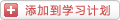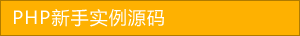﻿ 一起看看php实现购物车产品删除功能（2） - 综合实例 - PHP粉丝网

# 一起看看php实现购物车产品删除功能（2）

##### 发布:smiling　来源: PHP粉丝网 　添加日期：2022-07-12 08:43:25　浏览: 评论：0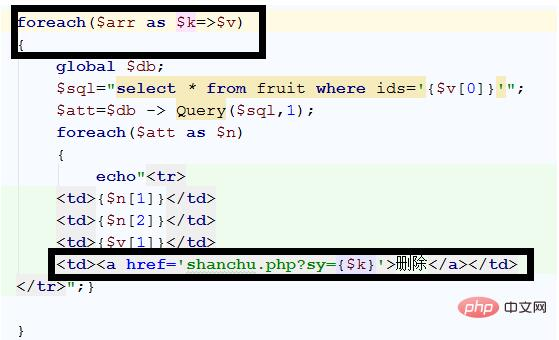<a href='shanchu.php?sy={\$k}'>删除</a></td>

1. <?php
2.
3. session_start();
4.
5. \$sy = \$_GET["sy"];
6.
7.
8.
9. //根据索引找到该数据
10.
11. \$arr = \$_SESSION["gwc"];
12.
13. \$arr[\$sy]; //要删除的数据
14.
15.
16.
17. //如果数量不为1，数量减1
18.
19. if(\$arr[\$sy]>1)
20.
21.
22.  \$arr[\$sy] = \$arr[\$sy]-1;
23.
24.
25. else //如果数量为1，移除
26.
27.
28.  unset(\$arr[\$sy]);
29.
30.
31.
32.
33. \$_SESSION["gwc"] = \$arr//最后存一下购物车的内容
34.
35.
36.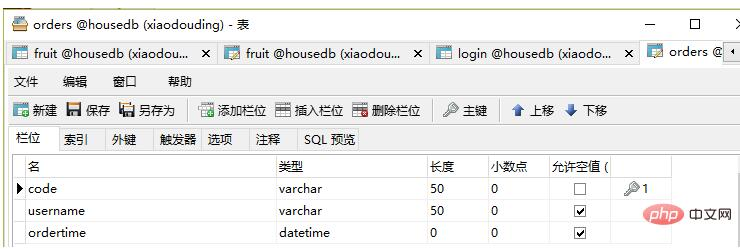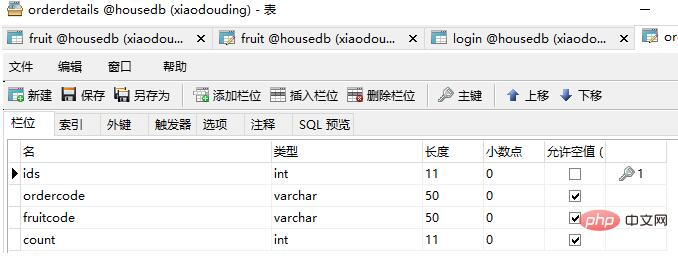<p><a href="tijiaodd.php" rel="external nofollow" ></a><p>

1. <?php
2.
3.  session_start();
4.
6.
7. \$uid=\$_SESSION["uid"];
8.
9. //先查一下账户余额
10.
12.
14.
15. \$ysql="select account from login where username='{\$uid}'"
16.
17. \$yarr=\$db->Query(\$ysql);
18.
19. \$yarr;//总额
20.
21.
22.
23.
24.
25. //购物车的总价格，前面有写过
26.
27.
28.
29. \$arr=array();
30.
31.
32.
33. if (!emptyempty(\$_SESSION["gwc"]))
34.
35.
36.  \$arr=\$_SESSION["gwc"];
37.
38.
39. \$sum=0;
40.
41. foreach(\$arr as \$v
42.
43.
44.  \$v;//购物车中产品的数量
45.
46.  \$psql="select price from fruit WHERE ids='{\$v}'"
47.
48.  \$parr=\$db->Query(\$psql);
49.
50.  foreach(\$parr as \$k
51.
52.  {
53.
54.   \$k;//产品的单价
55.
56.   \$sum+=\$k*\$v;
57.
58.  }
59.
60.
61.
62.
63. //判断余额是否满足购买
64.
65. if(\$yarr>=\$sum
66.
67. {//余额满足，要判断库存
68.
69.  foreach(\$arr as \$v
70.
71.  {
72.
73.   \$ksql="select number from fruit where ids='{\$v}'"
74.
75.   \$karr=\$db->Query(\$ksql);
76.
77.   \$karr;//这是库存
78.
79.   if(\$karr<\$v) //表示库存不足，这时要给顾客提示库存不足
80.
81.   {
82.
83.    echo"库存不足"
84.
85.    exit
86.
87.   }
88.
89.  }
90.
91.
92.
93.  //判断之后需要提交订单了
94.
95.  //账户扣除余额
96.
97.  \$kcsql="update login set account=account-{\$sum} where username='{\$uid}'"
98.
99.  \$db->Query(\$kcsql,0);//这里是修改语句，所以要加上0
100.
101.
102.
103.  //扣除库存
104.
105.  foreach(\$arr as \$v
106.
107.  {
108.
109.   \$kcksql="update fruit set number=number-\$v where ids='{\$v}'"
110.
111.   \$db->Query(\$kcksql,0);
112.
113.  }
114.
115.
116.
117. //所有的工作都做完了，这时我们就该提交订单了
118.
119. // 这里我在数据库中做了两张表，把提交的订单添加到表中就可以保存了
120.
121.
122.
123. //添加订单
124.
125. \$ddh = date("YmdHis");
126.
127. \$time = date("Y-m-d H:i:s");
128.
129. \$sdd = "insert into orders values('{\$ddh}','{\$uid}','{\$time}')"
130.
131. \$db->Query(\$sdd,0);
132.
133.
134.
135. //添加订单详情
136.
137.  foreach(\$arr as \$v
138.
139.  {
140.
141.   \$sddxq = "insert into orderdetails values('','{\$ddh}','{\$v}','{\$v}')"
142.
143.   \$db->Query(\$sddxq,0);
144.
145.  }
146.
147.
148.
149.
150. else
151.
152.
153.  echo "余额不足"
154.
155.  exit
156.
157.
158.
159.
160. ?>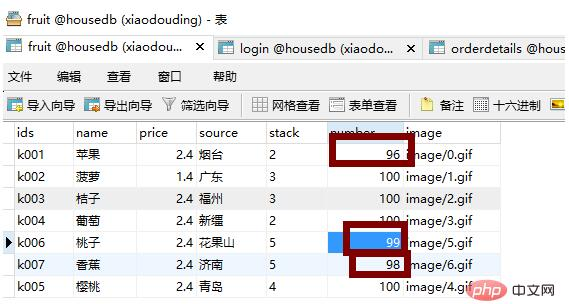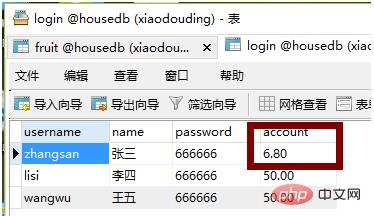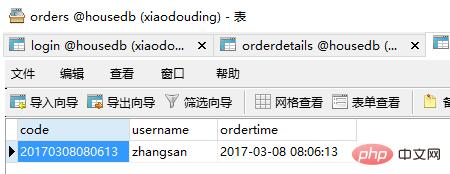### 分享到： document.write('<a href="http://v.t.sina.com.cn/share/share.php?url='+encodeURIComponent(location.href)+'&appkey=3172366919&title='+encodeURIComponent('一起看看php实现购物车产品删除功能（2）')+'" title="分享到新浪微博" class="t1" target="_blank">&nbsp;</a>'); document.write('<a href="http://www.douban.com/recommend/?url='+encodeURIComponent(location.href)+'&title='+encodeURIComponent('一起看看php实现购物车产品删除功能（2）')+'" title="分享到豆瓣" class="t2" target="_blank">&nbsp;</a>'); document.write('<a href="http://share.renren.com/share/buttonshare.do?link='+encodeURIComponent(location.href)+'&title='+encodeURIComponent('一起看看php实现购物车产品删除功能（2）')+'" title="分享到人人" class="t3" target="_blank">&nbsp;</a>'); document.write('<a href="http://www.kaixin001.com/repaste/share.php?rtitle='+encodeURIComponent('一起看看php实现购物车产品删除功能（2）')+'&rurl='+encodeURIComponent(location.href)+'&rcontent=" title="分享到开心网" class="t4" target="_blank">&nbsp;</a>'); document.write('<a href="http://sns.qzone.qq.com/cgi-bin/qzshare/cgi_qzshare_onekey?url='+encodeURIComponent(location.href)+'" title="分享到QQ空间" class="t5" target="_blank">&nbsp;</a>');